# Ernst Zermelo

Ernst Friedrich Ferdinand Zermelo (July 27, 1871, Berlin, German Empire – May 21, 1953, Freiburg im Breisgau, West Germany) was a German mathematician, whose work has major implications for the foundations of mathematics. His best know contribution is his axiomatization of set theory called Zermelo set theory, which later leads to Zermelo-Fraenkel set theory, the set theory that is now standard. Also he is the one who first explicitly formulated the axiom of choice.

## Life

Ernst Friedrich Ferdinand Zermelo was born on July 27 in 1871 at Berlin. He graduated from Berlin's Luisenstädtisches Gymnasium in 1889. He then studied mathematics, physics, and philosophy at the Universities of Berlin, Halle, and Freiburg. He finished his doctorate in 1894 at the University of Berlin, awarded for a dissertation on the calculus of variations (Untersuchungen zur Variationsrechnung). Zermelo remained at the University of Berlin, where he was appointed assistant to Planck, under whose guidance he began to study hydrodynamics. In 1897, Zermelo went to Göttingen, at that time the leading centre for mathematical research in the world, where he completed his habilitation thesis in 1899.

In 1900, in the Paris conference of the International Congress of Mathematicians, David Hilbert challenged the mathematical community with his famous Hilbert's problems, a list of 23 unsolved fundamental questions which mathematicians should attack during the coming century. The first of these, a problem of set theory, was the continuum hypothesis introduced by Cantor in 1878.

Zermelo began to work on the problems of set theory and in 1902 published his first work concerning the addition of transfinite cardinals (a kind of numbers to describe the size of infinity). In 1904, he succeeded in taking the first step suggested by Hilbert towards the continuum hypothesis when he proved the well-ordering theorem (every set can be well ordered). This result brought fame to Zermelo, who was appointed Professor in Göttingen, in 1905. His proof of the well-ordering theorem based on the axiom of choice, the first explicit formulation of which is credit to him, was not accepted by all mathematicians, partly because set theory was not axiomatized at this time. In 1908, Zermelo succeeded in producing a much more widely-accepted proof.

In 1905, Zermelo began to axiomatize set theory; in 1908, he published his results despite his failure to prove the consistency of his axiomatic system. (See below for the details of Zermelo's axiomatization.)

In 1922, Adolf Fraenkel and Thoralf Skolem independently improved Zermelo's axiom system. The resulting 10 axiom system, now called 'Zermelo–Fraenkel set theory (ZF), is now the most commonly used system for axiomatic set theory.

In 1910, Zermelo left Göttingen upon being appointed to the chair of mathematics at Zurich University, which he resigned in 1916. He was appointed to an honorary chair at Freiburg im Breisgau in 1926, which he resigned in 1935 because he disapproved of Hitler's regime. At the end of World War II and at his request, Zermelo was reinstated to his honorary position in Freiburg. He died in 1953.

## Zermelo Set Theory

In 1908, Zermelo published an important paper where he pushed forward the axiomatization of set theory, now called 'Zermelo set theory. The axioms of the system, with the original text (translated into English) and original numbering, are as follows:

AXIOM I. Axiom of extensionality (Axiom der Bestimmtheit) "If every element of a set M is also an element of N and vice versa ... then M = N. Briefly, every set is determined by its elements."
AXIOM II. Axiom of elementary sets (Axiom der Elementarmengen) "There exists a (fictitious) set, the null set, ∅, that contains no element at all. If a is any object of the domain, there exists a set {a} containing a and only a as element. If a and b are any two objects of the domain, there always exists a set {a, b} containing as elements a and b but no object x distinct from them both."
AXIOM III. Axiom of separation (Axiom der Aussonderung) "Whenever the propositional function –(x) is definite for all elements of a set M, M possesses a subset M'  containing as elements precisely those elements x of M for which –(x) is true."
AXIOM IV. Axiom of the power set (Axiom der Potenzmenge) "To every set T there corresponds a set T' , the power set of T, that contains as elements precisely all subsets of T."
AXIOM V. Axiom of the union (Axiom der Vereinigung) "To every set T there corresponds a set ∪T, the union of T, that contains as elements precisely all elements of the elements of T."
AXIOM VI. Axiom of choice (Axiom der Auswahl): "If T is a set whose elements all are sets that are different from ∅ and mutually disjoint, its union ∪T includes at least one subset S1 having one and only one element in common with each element of T."
AXIOM VII. Axiom of infinity (Axiom des Unendlichen) "There exists in the domain at least one set Z that contains the null set as an element and is so constituted that to each of its elements a there corresponds a further element of the form {a}, in other words, that with each of its elements a it also contains the corresponding set {a} as element."

## Zermelo-Fraenkel Set Theory

Zermelo's axiomatic theory had some defects. First, it did not allow the construction of the ordinal numbers; while most of "ordinary mathematics" can be developed without ever using ordinals, ordinals are an essential tool in most set-theoretic investigations. Second, one of Zermelo's axioms invoked a concept, that of a "definite" property, whose operational meaning was ambiguous.

In 1922, Abraham Fraenkel and Thoralf Skolem independently proposed defining a "definite property" as any property that could be formulated in first-order logic, such that all atomic formulae involve set membership or equality. Also from their work emerged the axiom of replacement, which allows the construction of ordinal numbers. Appending this axiom, as well as the axiom of regularity, to Zermelo set theory yields Zermelo-Fraenkel set theory by ZF.

ZF has the following axioms:

1) Axiom of extensionality: Two sets are the same if they have the same elements.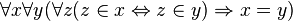$\forall x \forall y ( \forall z (z \in x \Leftrightarrow z \in y) \Rightarrow x = y)$

The converse of this axiom is a consequence of the substitution property of equality.

2) Axiom of regularity (also called the Axiom of foundation): Every non-empty set x contains some member y such that x and y are disjoint sets.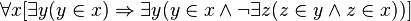$\forall x [ \exists y ( y \in x) \Rightarrow \exists y ( y \in x \land \lnot \exists z (z \in y \land z \in x))]$

3) Axiom schema of specification (also called the Axiom schema of separation or the Axiom schema of restricted comprehension): If z is a set, and$\phi\!$ is any property which may characterize the elements x of z, then there is a subset y of z containing those x in z which satisfy the property. The restriction to z is necessary to avoid Russell's paradox and its variants. More formally, let$\phi\!$ be any formula in the language of ZF, and let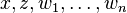$x,z,w_1,\ldots,w_n\!$ be free variables appearing in$\phi\!$. Then: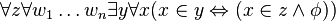$\forall z \forall w_1 \ldots w_n \exists y \forall x (x \in y \Leftrightarrow ( x \in z \land \phi ) )$

Specification is part of Z but redundant in ZF, because there are variants of ZF's axiom schema of replacement that turn Specification into a theorem.

4) Axiom of pairing: If x and y are sets then there exists a set containing both of them.$\forall x \forall y \exist z (x \in z \land y \in z)$

Pairing is part of Z but is redundant in ZF, because ZF's axiom schema of replacement (in combination with the axiom of infinity turns Pairing into a theorem. Also the axiom of pairing with the axiom of extensionality yields Axiom II in Zermelo set theory.

5) Axiom of union: For any set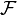$\mathcal{F}$ there is a set A containing every set that is a member of some member of$\mathcal{F}$.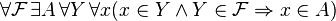$\forall \mathcal{F} \,\exists A \, \forall Y\, \forall x (x \in Y \land Y \in \mathcal{F} \Rightarrow x \in A)$

6) Axiom schema of replacement: Informally, if the domain of a function f is a set, then the range of f is also a set, subject to a restriction to avoid paradoxes. Formally, let the formula$\phi \!$ and its free variables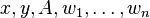$x,y,A,w_1,\ldots,w_n \!$ be as described in the axiom schema of specification. Then: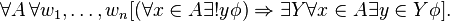$\forall A\,\forall w_1,\ldots,w_n [ ( \forall x \in A \exists ! y \phi ) \Rightarrow \exists Y \forall x \in A \exists y \in Y \phi].$

Here the quantifer$\exists ! y$ means that only one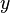$y\!$ exists, up to equality.

The next axiom employs the notation$S(x) = x \cup \{x\} \!$, where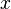$x \!$ is some set. From axioms 1 through 6 above, the existence and uniqueness of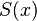$S(x)\!$ and of the empty set can be proved. The latter fact makes redundant Kunen's axiom (not shown) asserting the existence of at least one set.

7) Axiom of infinity: There exists a set X such that the empty set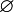$\varnothing$ is a member of X and whenever y is in X, so is S(y).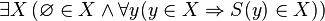$\exist X \left (\varnothing \in X \and \forall y (y \in X \Rightarrow S(y) \in X)\right )$

8) Axiom of power set: For any set x there is a set y that contains every subset of x.$\forall x \exists y \forall z (z \subseteq x \Rightarrow z \in y)$

Here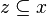$z \subseteq x$ is an abbreviation for$\forall q (q \in z \Rightarrow q \in x)$.

The system called ZFC is obtained by adding to ZF the axiom of choice:

9) Axiom of choice: For any set X there is a binary relation R which well-orders X. This means that R is a linear order on X and every nonempty subset of X has an element which is minimal under R.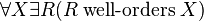$\forall X \exists R ( R \;\mbox{well-orders}\; X)$

The axiom of choice has many equivalent statements; that is, there are many statements that can be proved equivalent to axiom 9 using axioms 1-8. The name "axiom of choice" refers to one such statement, namely that there exists a choice function for every set of nonempty sets. Since the existence of a choice function for finite sets is easily proved, this formulation is interesting because of what it asserts about certain infinite sets.

There are many equivalent formulations of the ZFC axioms; for a rich but somewhat dated discussion of this fact, see Fraenkel et al. (1973). The above particular axiom set is that of Kunen (1980).